Search:

Ammonia is added progressively (from left to right) to a copper(II) chloride solution:The things are quite complicated. The two "fathers" of the theory of complexes, Alfred Werner and Sophus Mads Jorgensens have quarelled for twenty years about the structure of the complex ions produced during this addition. Anyway, the green color corresponds to $[Co(NH_3)_4Cl_2]^+$ (see the charge!!), the yellow color to $[Co(NH_3)_6]^{3+}$ (see the charge!!) The ligands $NH_3$ et $Cl^-$ make the coordination sphere. The coordination number is the number of ligands, here always 6.

## The ligands

Here are the names of some current ligands: In brackets the new. sparely accepted nomenclature !

Ligands

 $H_2O$ aqua $OH^-$ hydroxo $NH_3$ ammine $CN^-$ cyan(id)o $SCN^-$ thiocyan(id)o $NO_2^-$ nitr(it)o $F^-$ fluor(id)o $Cl^-$ chlor(id)o $Br^-$ brom(id)o $I^-$ iod(id)o $S_2O_3^{2-}$ thiosulfato

The number of ligands is indicated by the prefixes

Prefixes di, tri, tetra, penta, hexa, hepta, octo, nona, deca, undeca, dodeca or bis, tris, tetrakis, pentakis,... often used in case of long ligand names.

The global charge of the complex is the sum of the charge of the central positive ion and the negative ligands. - If the global charge of the complex is positive or zero, the central atom keeps its normal name - If it is negative, the central atom takes one of following names:

Anions

 $Pb$ plumbate $Cu$ cuprate $Au$ aurate $Al$ aluminate $Ag$ argentate $Fe$ ferrate $Zn$ zincate $Ni$ nickelate $Co$ cobaltate $Hg$ mercurate $Sn$ stannate

The charge of the central ion is often indicated by latin numbers: $[Cu(H_2O)_5]^{2+}$ pentaaqua copper(II) $[Cu(CN)_4]^{2-}$ tetracyan(id)o cuprate(II) $[$A$g(NH_3)_2]^{+}$ diammine silver(I) $[Co(NO_2)_6]^{3-}$ hexanitr(it)o cobaltate(III) Normally anions come first in the formula, for other ligands the alphabetic order is indicated: $[CoCl_2(NH_3)_4]^{+}$ dichlor(id)o tetraammine cobalt(III) $[Ni(NH_3)_5(H_2O)]^{2+}$ pentaammine aqua nickel(II)

## The strength of the ligands

Ligands which are weakly bound to the central ion ("weak" ligands) can be displaced by "strong" ligands Concentrated chlorhydric acid is added gradually (from left to right) to a copper(II) sulfate solution: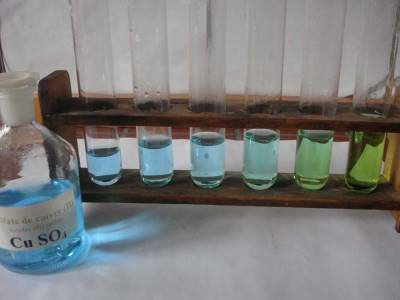$[Cu(H_2O)_5]^{2+}$ (blue) is gradually transformed to $[CuCl_4]^{2-}$ (greenish-yellow) because the chloride ions liberated by the chlorhydric acid are stronger ligands than the water molecules. A solution of potassium thiocyanate is added to an iron(III) chloride solution: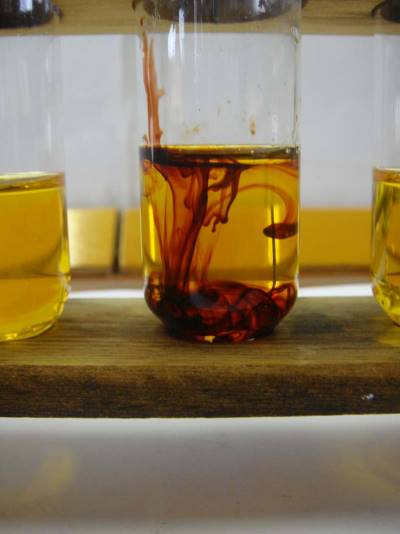The complex ion $[Fe(SCN)]^{2+}$ with a blood red color is produced. Now a solution of sodium fluoride is added (from left to right):$[Fe(SCN)]^{2+}$(blood red) is discoloured beeing transformed to the colourless $[FeF_6]^{3-}$ because the fluoride ions are stronger ligands then the thiocyanate ions.

## The chelates

Some ligands have more than one negative ($-$) or partially negative ($\delta -$) attachment point. They catch the central ion like a lobster catching a prey with their claws (chelicerae). The coordination complex formed is then called chelate: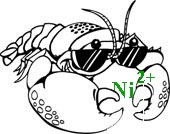The 1,2-diaminoethane (trivial name: ethylenediamine) $H_2N-CH_2-CH_2-NH_2$ has partially negative charges $\delta -$ on its nitrogen atom by which it attachs to $Ni^{2+}$ :Tris(ethylenediamino)nickel(II) Many important biochemical molecules are chelates, for instance :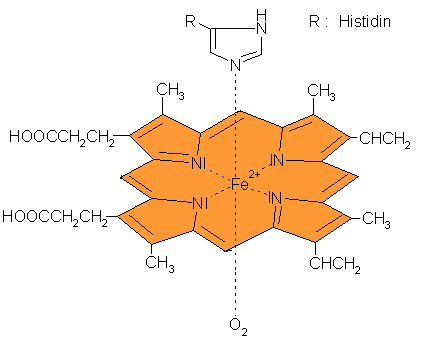Hemoglobine or: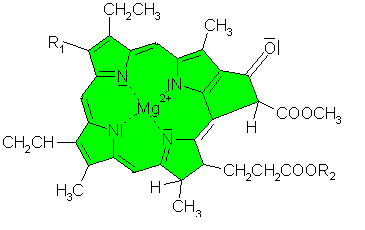Chlorophyll